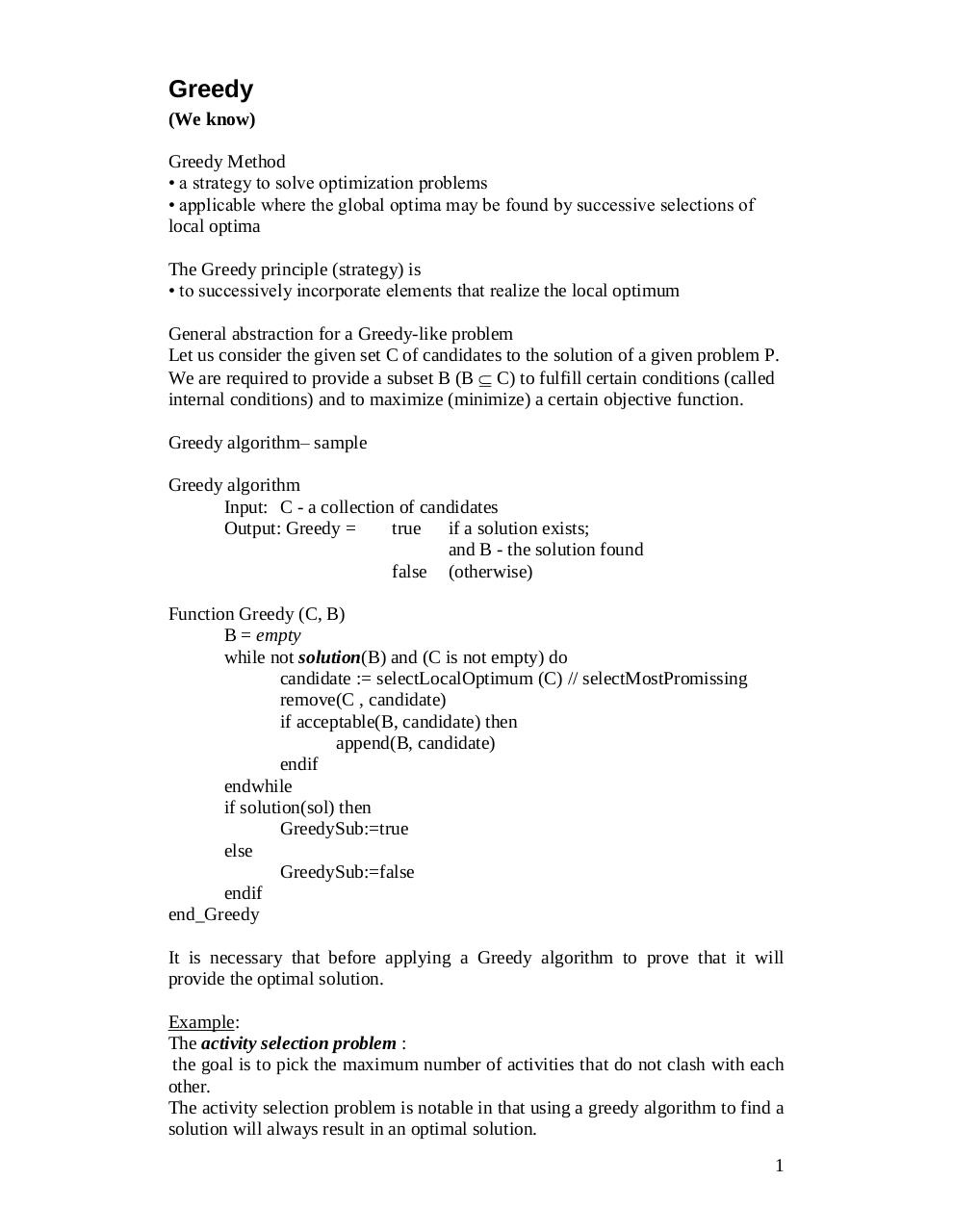# Lecture04.pdfPage 1 2 3 4 5 6

#### Text preview

Greedy
(We know)
Greedy Method
• a strategy to solve optimization problems
• applicable where the global optima may be found by successive selections of
local optima
The Greedy principle (strategy) is
• to successively incorporate elements that realize the local optimum
General abstraction for a Greedy-like problem
Let us consider the given set C of candidates to the solution of a given problem P.
We are required to provide a subset B (B  C) to fulfill certain conditions (called
internal conditions) and to maximize (minimize) a certain objective function.
Greedy algorithm– sample
Greedy algorithm
Input: C - a collection of candidates
Output: Greedy =
true if a solution exists;
and B - the solution found
false (otherwise)
Function Greedy (C, B)
B = empty
while not solution(B) and (C is not empty) do
candidate := selectLocalOptimum (C) // selectMostPromissing
remove(C , candidate)
if acceptable(B, candidate) then
append(B, candidate)
endif
endwhile
if solution(sol) then
GreedySub:=true
else
GreedySub:=false
endif
end_Greedy
It is necessary that before applying a Greedy algorithm to prove that it will
provide the optimal solution.
Example:
The activity selection problem :
the goal is to pick the maximum number of activities that do not clash with each
other.
The activity selection problem is notable in that using a greedy algorithm to find a
solution will always result in an optimal solution.
1